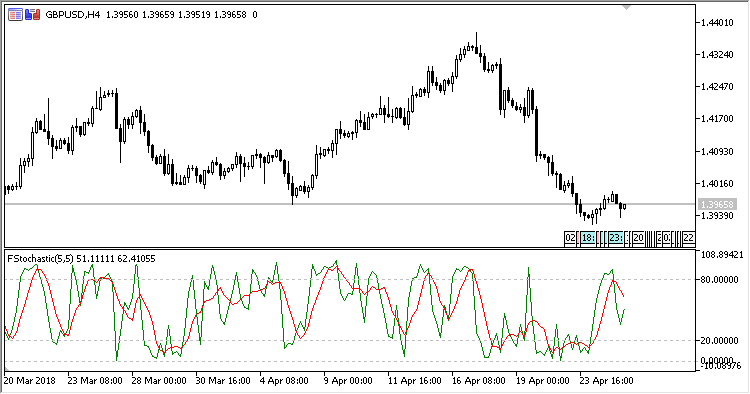# Stochastic_Fast – indicator for MetaTrader 5

• A+
category：MT5 INDICATORS

The Fast Stochastic oscillator shows in a separate window a stochastic calculated as follows:

`FSK[i] = (min / max) * 100.0,`
`FSD[i] = SMA(FSK,PeriodD)`

where:

```min = close[i] - low[BL],
max = high[BH] - low[BL],
BH is the highest price index within the range from PeriodK to i,
BL is the lowest price index within the range from PeriodK to i,
```

if max = 0,

`FSK[i] = 50.0`

The indicator has four input parameters:

• Period K - the period of calculating line K;
• Period D - the period of calculating line D;
• Overbought - overbought level;
• Oversold - oversold level.